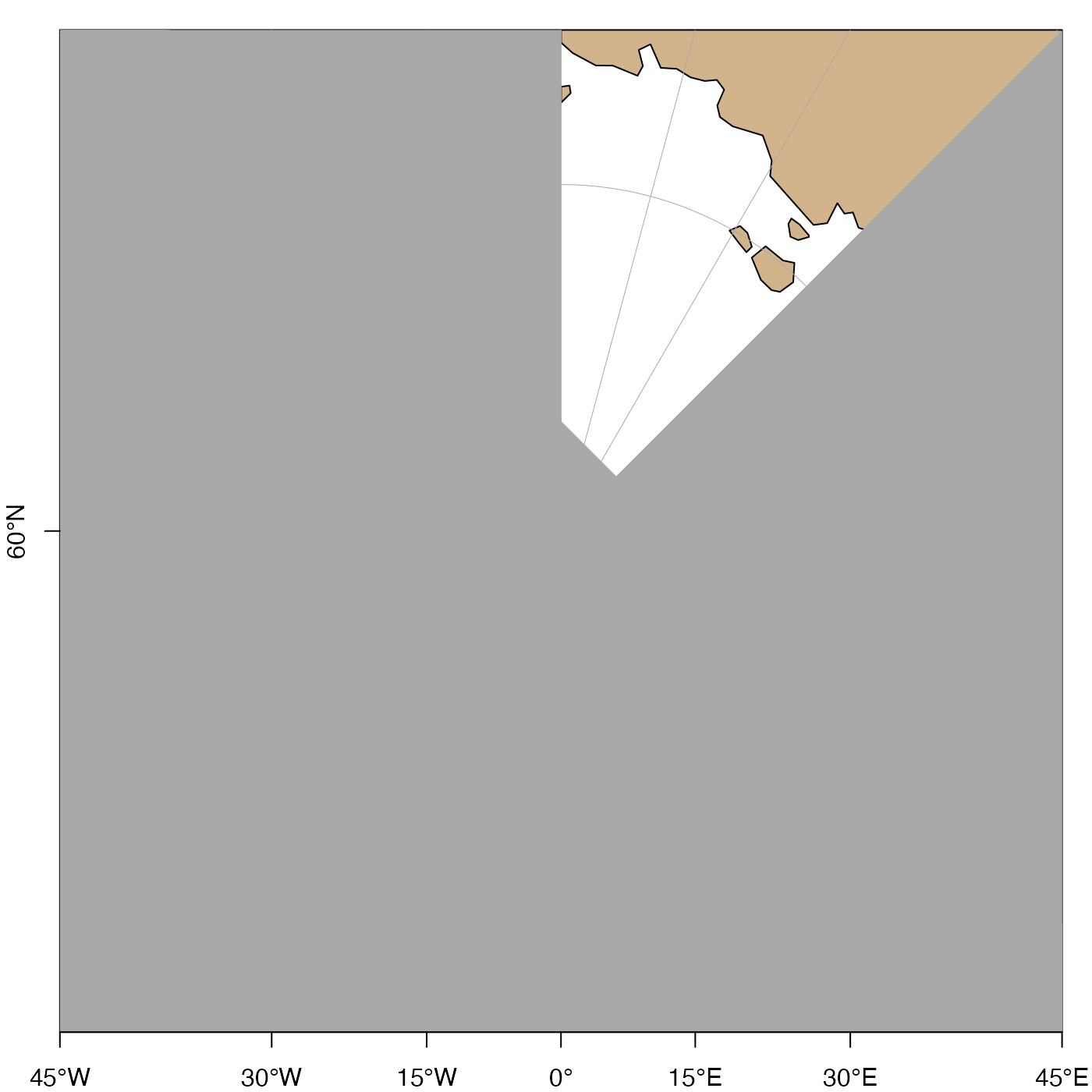Plot axis labels on an existing map.

mapAxis(
side = 1:2,
longitude = TRUE,
latitude = TRUE,
axisStyle = 1,
tick = TRUE,
line = NA,
pos = NA,
outer = FALSE,
font = NA,
lty = "solid",
lwd = 1,
lwd.ticks = lwd,
col = NULL,
col.ticks = NULL,
tcl = -0.3,
cex.axis = 1,
mgp = c(0, 0.5, 0),
debug = getOption("oceDebug")
)

## Arguments

side the side at which labels are to be drawn. If not provided, sides 1 and 2 will be used (i.e. bottom and left-hand sides). either a logical value or a numeric vector of longitudes. There are three possible cases: (1) If longitude=TRUE (the default) then ticks and nearby numbers will occur at the longitude grid established by the previous call to mapPlot(); (2) if longitude=FALSE then no longitude ticks or numbers are drawn; (3) if longitude is a vector of numerical values, then those ticks are placed at those values, and numbers are written beside them. Note that in cases 1 and 3, efforts are made to avoid overdrawing text, so some longitude values might get ticks but not numbers. To get ticks but not numbers, set cex.axis=0. similar to longitude but for latitude. an integer specifying the style of labels for the numbers on axes. The choices are: 1 for signed numbers without additional labels; 2 (the default) for unsigned numbers followed by letters indicating the hemisphere; 3 for signed numbers followed by a degree sign; 4 for unsigned numbers followed by a degree sign; and 5 for signed numbers followed by a degree sign and letters indicating the hemisphere. parameter passed to axis(). parameter passed to axis(). parameter passed to axis(). parameter passed to axis(). axis font, passed to axis(). axis line type, passed to axis(). axis line width, passed to axis()). tick line width, passed to axis(). axis color, passed to axis(). axis tick color, passed to axis(). an argument that is transmitted to axis(). an argument that is transmitted to axis(). axis-tick size (see par()). axis-label expansion factor (see par()); set to 0 to prevent numbers from being placed in axes. three-element numerical vector describing axis-label placement (see par()). It usually makes sense to set the first and third elements to zero. a flag that turns on debugging. Set to 1 to get a moderate amount of debugging information, or to 2 to get more.

## Details

This function is still in development, and the argument list as well as the action taken are both subject to change, hence the brevity of this help page.

Note that if a grid line crosses the axis twice, only one label will be drawn.

A map must first have been created with mapPlot().

Other functions related to maps: formatPosition(), lonlat2map(), lonlat2utm(), map2lonlat(), mapArrows(), mapContour(), mapCoordinateSystem(), mapDirectionField(), mapGrid(), mapImage(), mapLines(), mapLocator(), mapLongitudeLatitudeXY(), mapPlot(), mapPoints(), mapPolygon(), mapScalebar(), mapText(), mapTissot(), oceCRS(), shiftLongitude(), usrLonLat(), utm2lonlat()

## Examples

# \donttest{
library(oce)
data(coastlineWorld)
par(mar=c(2, 2, 3, 1))
lonlim <- c(-180, 180)
latlim <- c(60, 120)
mapPlot(coastlineWorld, projection="+proj=stere +lat_0=90",
longitudelim=lonlim, latitudelim=latlim,
grid=FALSE)mapGrid(15, 15, polarCircle=1/2)mapAxis()#> Warning: mapAxis(side=1) cannot draw latitude labels yet; contact author if you need this#> Warning: mapAxis(side=2) cannot draw longitude labels yet; contact author if you need this# }